4068

Physics Circular Motion Level: Misc Level

Find the angular speed of the earth about the sun in radians per second ad degrees per day. (mean radius=6.38*10^6 m) (distance from sun=1.496x10^11 m) (Period earth =3.156*10^7 sec)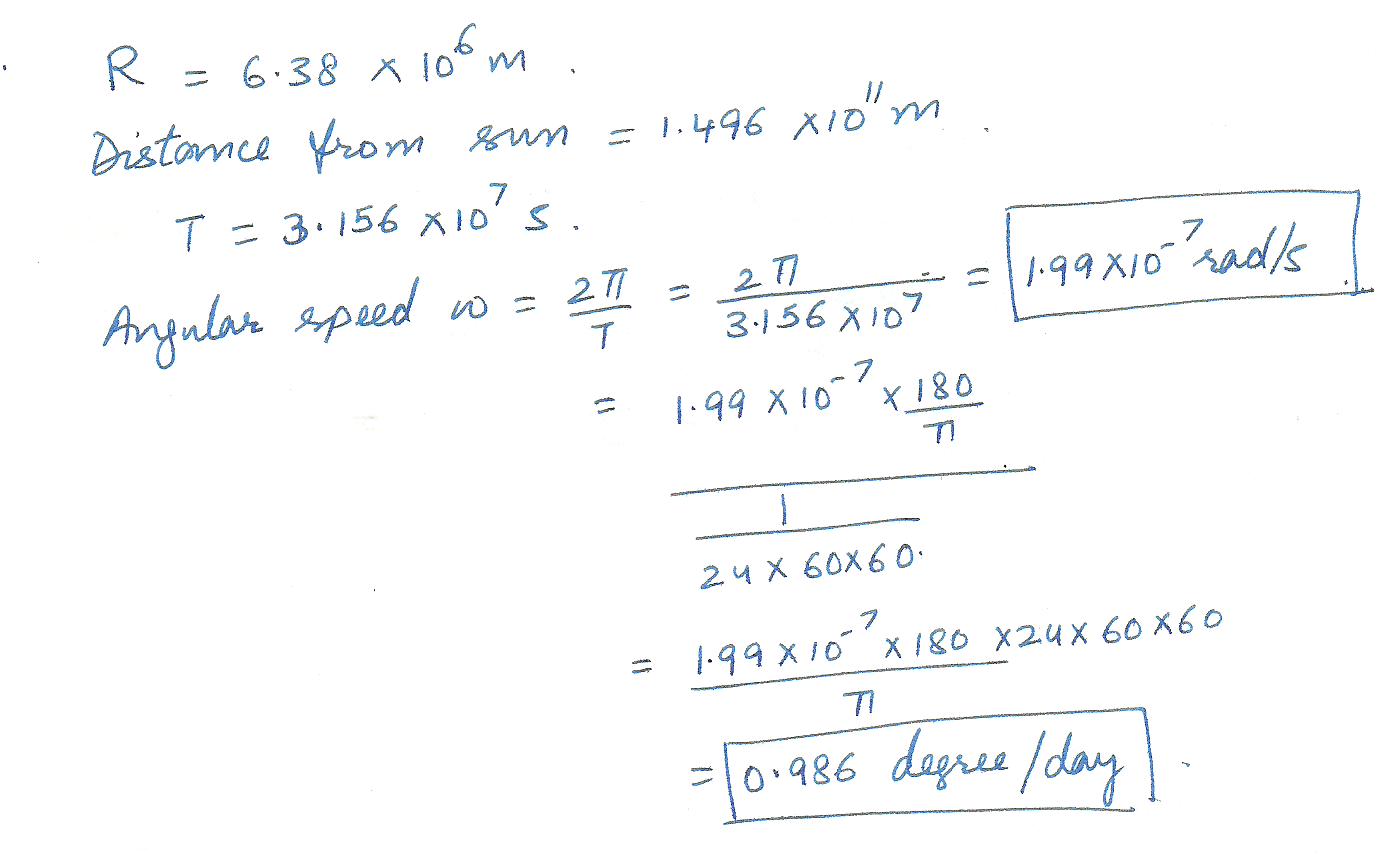4054

Physics Circular Motion Level: Misc Level

8. A dentist causes the bit of a high - speed drill to accelerate from an angular speed of 1.45*104 rad/s to an angular speed of 3.14*104 rad/s. In the process, the bit turns through 1.88*104 rad. Assuming a constant angular acceleration. how long would it take the bit to reach its maximum speed of 7.85 *104 rad/s, starting from rest?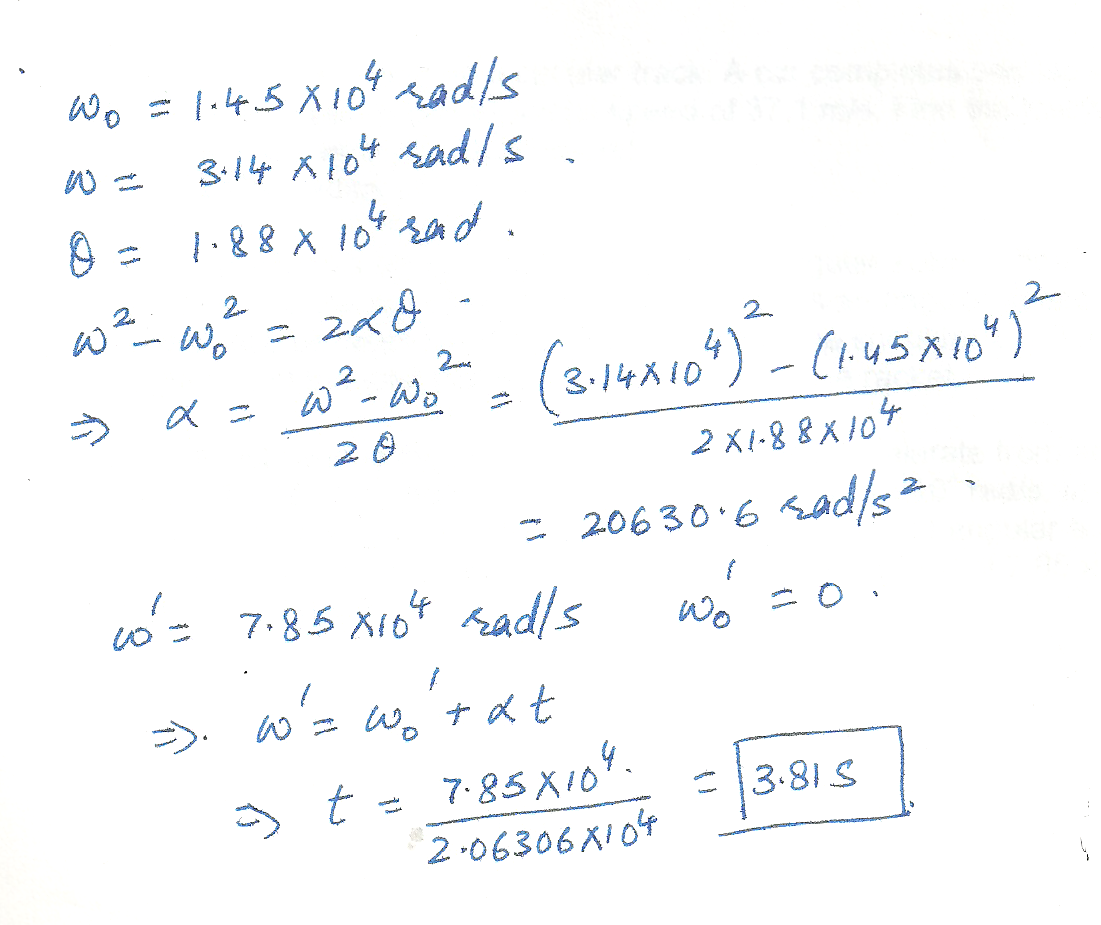4052

Physics Circular Motion Level: Misc Level

6. An auto race is held on a circular track. A car completes one lap in a time of 17.5 s, with an average tangential speed of 37. 1 m/s. Find the following.

a) the average angular speed

b) the radius of the track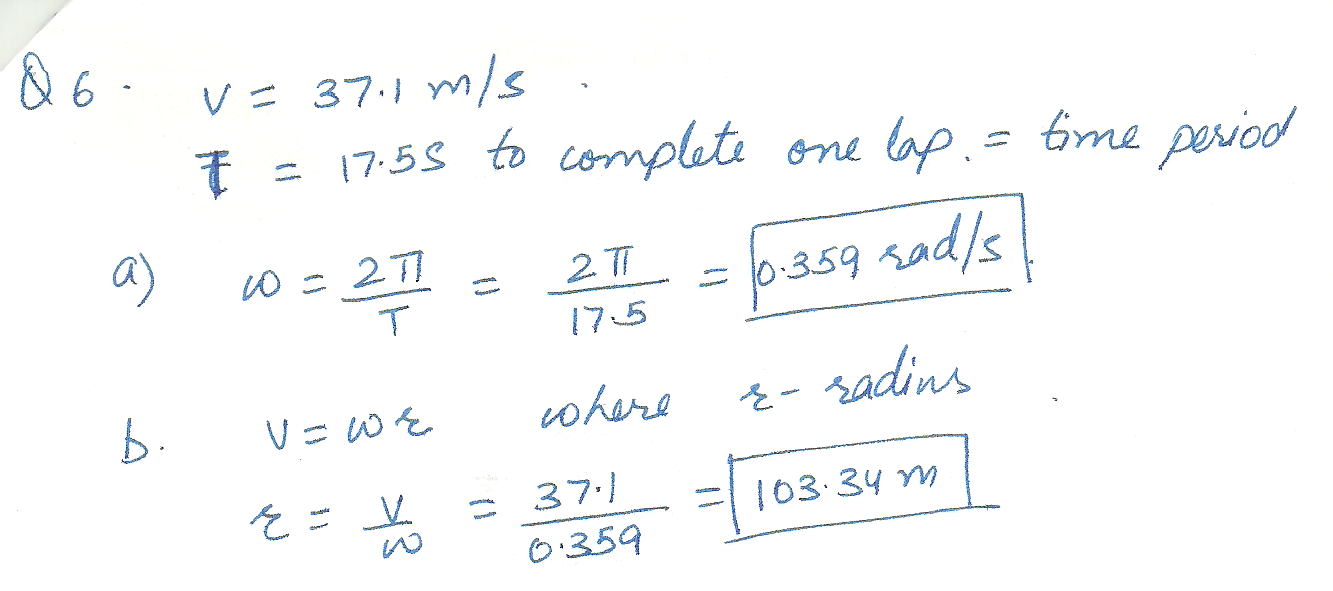4051

Physics Circular Motion Level: Misc Level

5. At the local swimming hole, a favorite trick is to run horizontally off a cliff that is 5.3 m above the water. One diver runs off the edge of the cliff, tucks into a " ball," and rotates on the way down with an average angular speed of 1.8 rev/s. Ignore air resistance and determine the number of revolutions she makes while on the way down.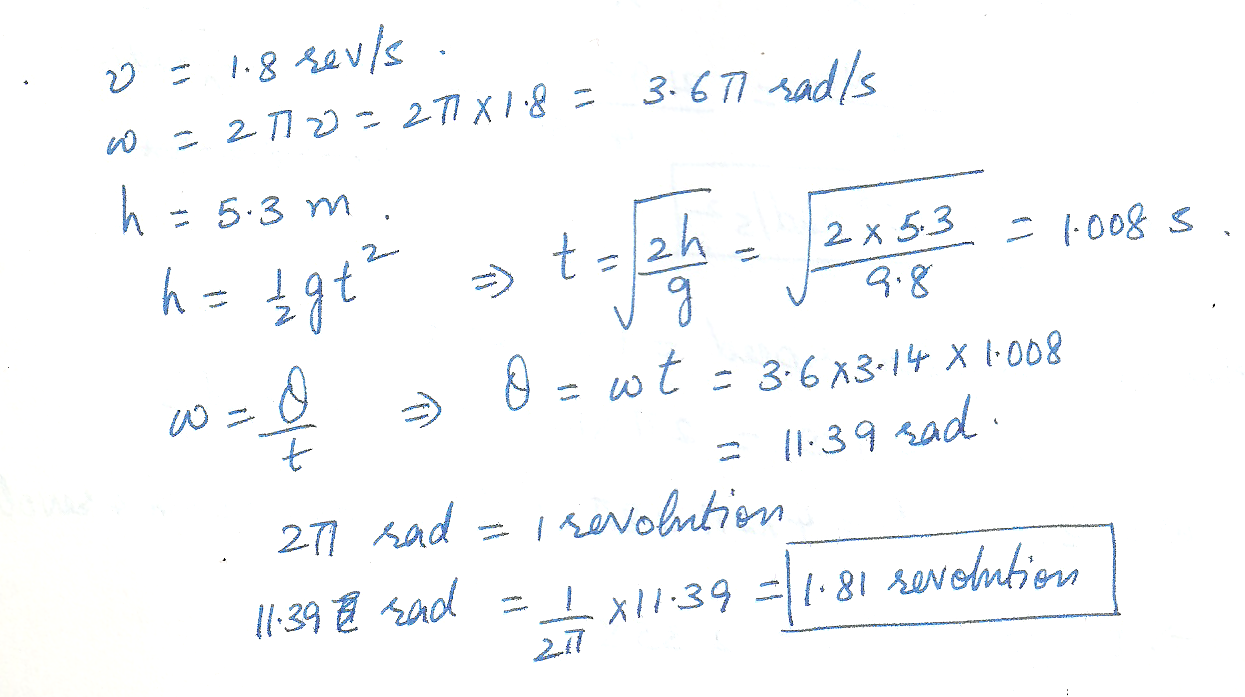4050

Physics Circular Motion Level: Misc Level

4. A person is riding a bicycle, and its wheels have an angular velocity of +24.5 rad/s. Then, the brakes are applied and the bike is brought to a uniform stop.During braking, the angular displacement of each wheel is +15.5 revolutions.

a) How much time does it take for the bike to come to rest?

b) What is the angular acceleration of each wheel?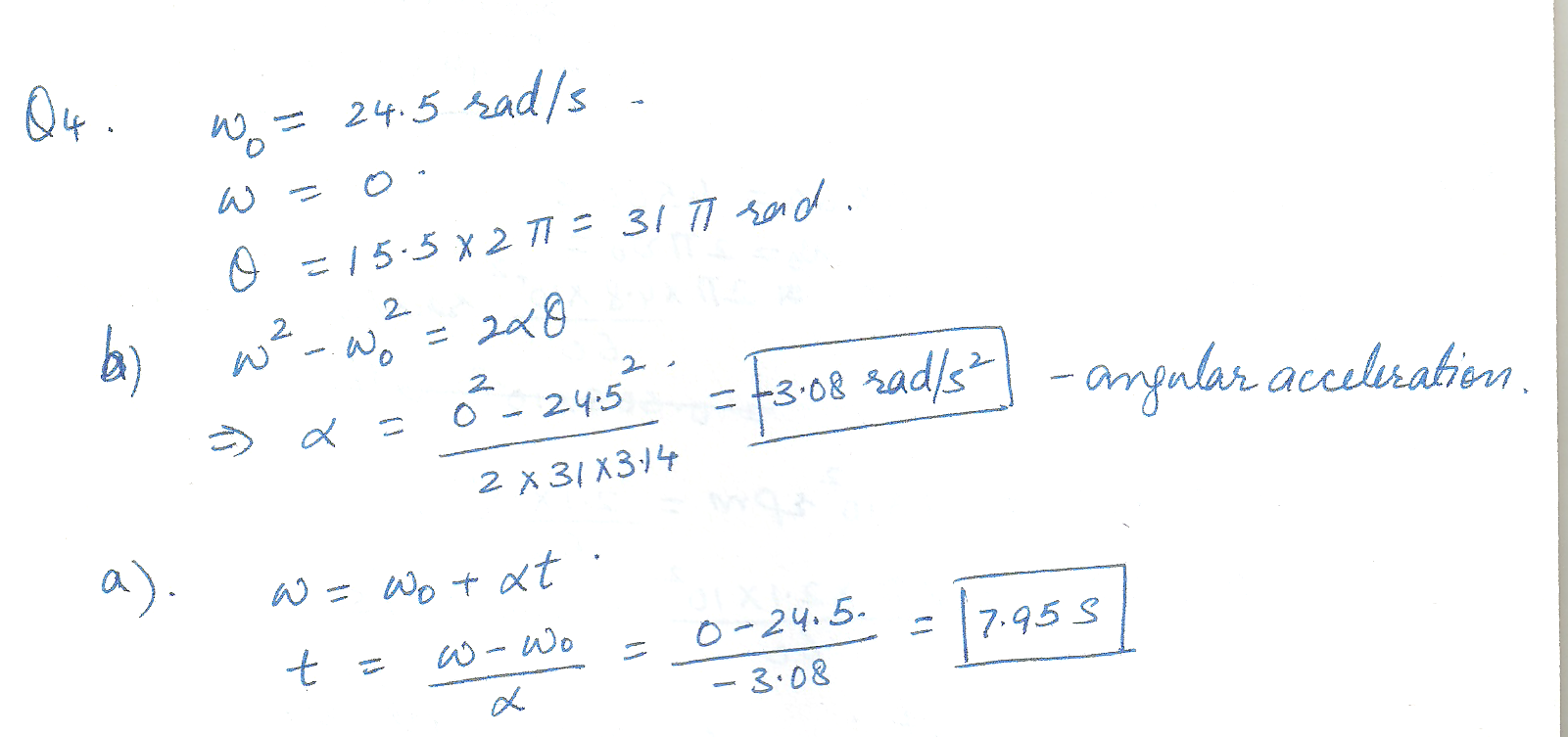4049

Physics Circular Motion Level: Misc Level

2. A certain CD has playing time of 76 minutes. When the music stars, the CD is rotating at an angular speed of 4.8* 102 revolutions per minute (rpm). At the end of the music, the CD is rotating at 2.1* 102 rpm. Find the magnitude of the average angular acceleration of the CD. Express your answer in rad/s 2.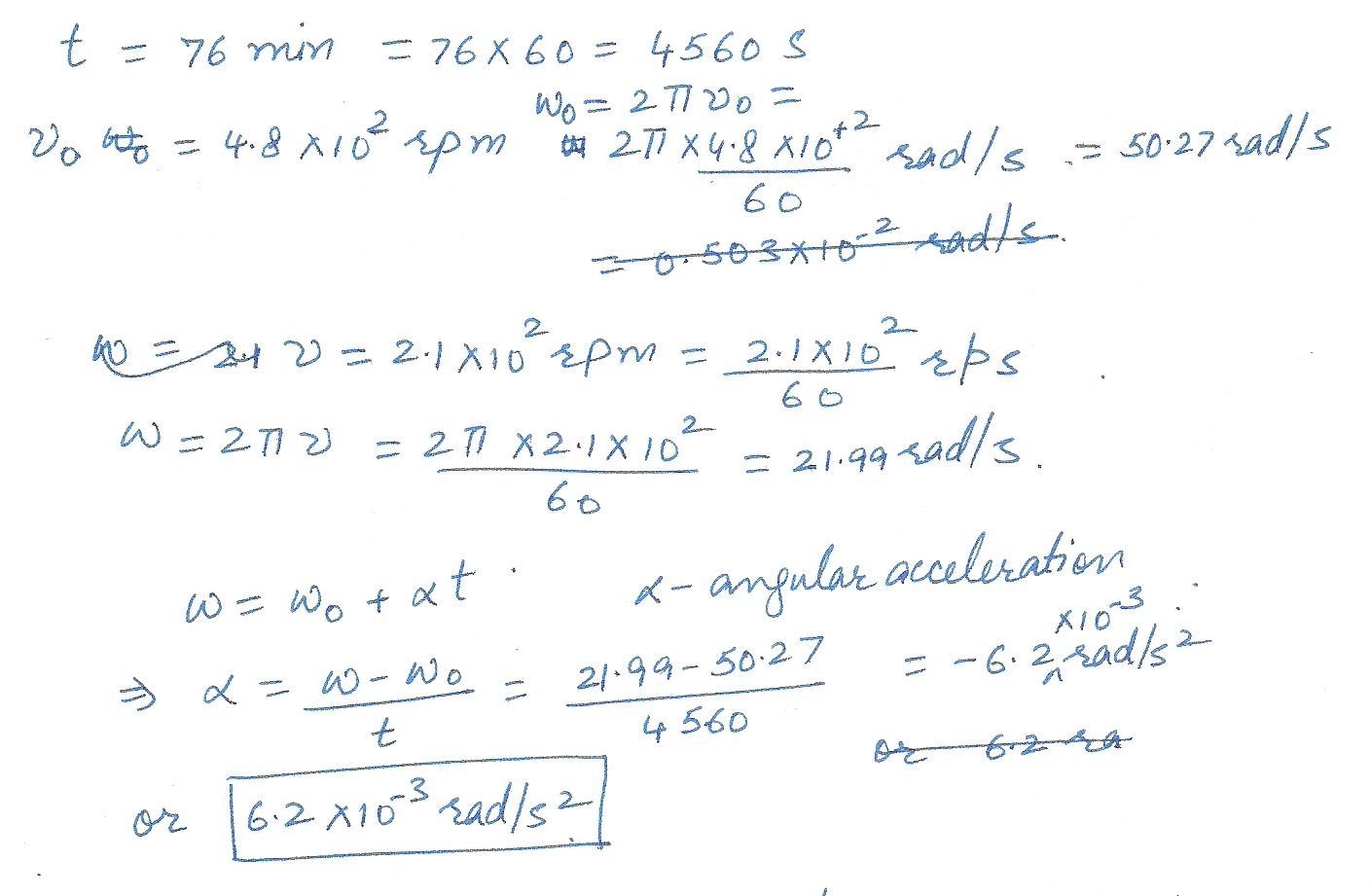4048

Physics Circular Motion Level: Misc Level

1. The earth spins on its axis once a day and orbits the sun once a year (365. 24 days).Take the positive direction for the angular displacement to be the direction of the earth s motion.

a) Determine the average angular velocity of the earth as it spins on its axis.

b) Determine its angular velocity as it revolves around the sun.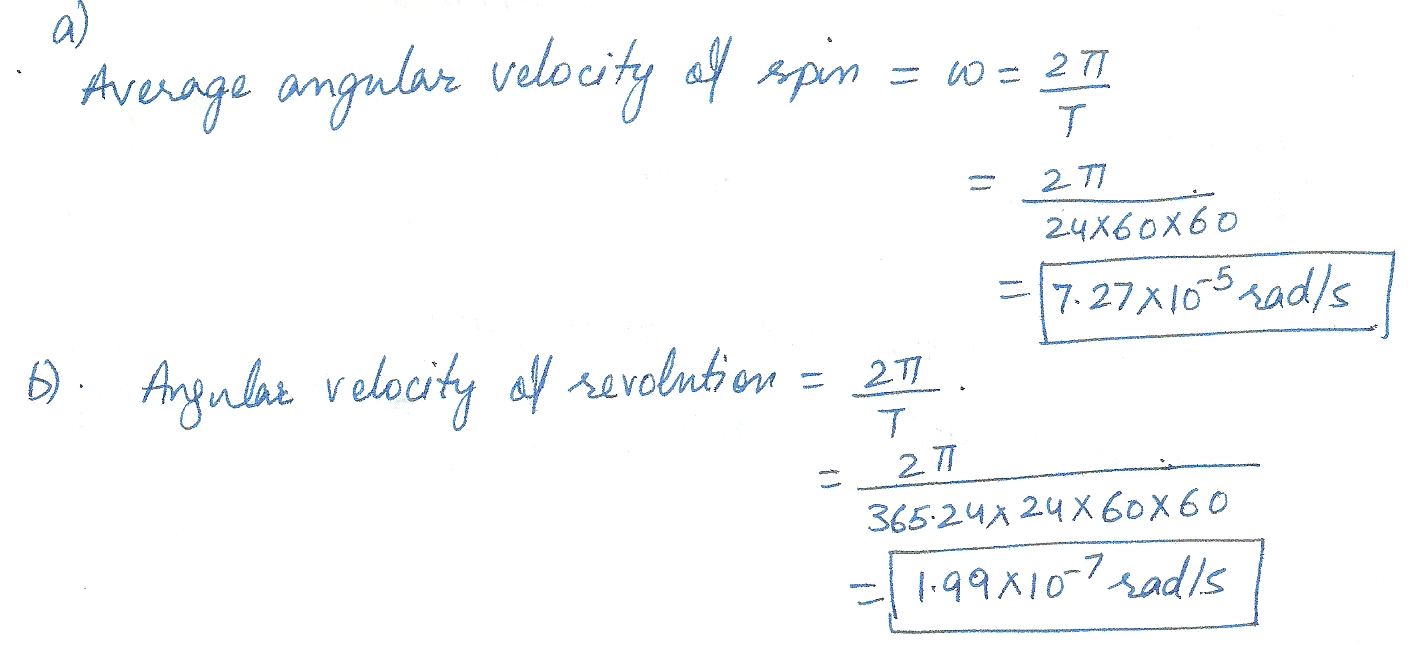Displaying 46-52 of 52 results.